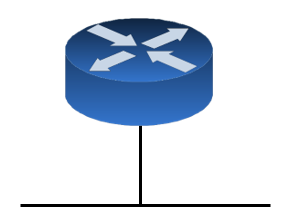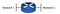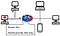# How to quickly subnet IP addresses

This article aims to help computer networking students, IT Professionals, or individuals interested in computer networking to be able to subnet IP addresses in under 20 seconds (usually) and perform the calculation purely in their head as opposed to googling “is 192.168.1.24 inside the 192.168.1.0/128 network” or using an online tool such as subnet calculator. We will only be dealing with IPV4 in this guide. Whether to impress your manager for a raise, find an endpoint quickly in a network, or to have a quicker security investigations using an SIEM tool, this technique would help a lot in your career.Fig 1. All devices under one network — All devices under one network: 192.168.0.0 to 192.168.255.255.

Historically, IP addresses were only of class A, B, C, and D for multicast ip address but today we have “classless” ip addressing. The figure on the right shows a router with only a single network.Fig 2. The same network in figure 1 but is divided into two — Network 1 ranges from 192.168.0.0 to 192.168.127.255 while Network 2 is 192.168.128.0 to 192.168.255.255.

Suppose we have a class C network:192.168.0.0 to 192.168.255.255 and want to divide the network by two.

Subnetting is a way to divide our class C Network (192.168.0.0 to 192.168.255.255) into 2 or more parts (in this case, divide our network in 2 parts like figure 2) such that the network becomes: 192.168.0.0 to 192.168.127.255 (Network 1) and 192.168.128.0 to 192.168.255.255 (Network 2).

What we are doing does not follow the standard classful IP addressing. Classless IP addressing is very common today in enterprise networks and are quite rare in home networks. While subnetting is synonymous to CIDR (Classless inter-domain routing), CIDR is just IP addressing that is not following the class A, B, C, and D (hence the name classless) addressing while subnetting is the calculation done to ensure IP addressing is correct which will allow networking devices route packets to their proper destinations. CIDR’s purpose is to save unused IP addresses by having a preferable amount of IP addresses on a subnetwork and to limit the size of routing tables in routers.

So why bother dividing the network? Why not just put every computer and endpoint under one network like our original class C Network in Figure 1, make our lives easier, and do none of that subnetting stuff. If we have every thing under one network, if you’re familiar with broadcast packets, that is when a computer sends a broadcast packet (destination ip: 192.168.255.255 or 255.255.255.255), it would be received by every device on the network, from the CEO’s laptop to the noisy annoying coworker. Now what happens if not one computer sends a broadcast packet but 50 including smartphones, IOT devices, and printers? network congestion could happen possibly bringing down the network, “delaying” normal packets from being received, and wasting precious cpu resources not only on endpoints but also for switches.

While if we had divided our network into two, broadcast packets will only be forwarded to endpoints of the same network, minimizing the amount of broadcast packets received by endpoints by preventing it from traveling to the other network.

Other advantages to CIDR are to protect the network against lateral movement by hackers, an example of this is if a hacker has infected and taken control of a computer with an IP address of 192.168.0.5 and wants to “hop” to the CEO’s computer with an IP address of 192.168.128.5. If we had not divided our network into two through subnetting, the hacker could simply RDP, Telnet, or SSH (if the services are installed and enabled on the target) to the CEO’s computer 192.168.128.5.Fig 5. Similar to the broadcast packet being stopped by the router in figure 4 — The router blocks the RDP from the infected system beause the router’s ACL allows port HTTP and HTTPS traffic through the interface.

Now if we have divided our network into two through subnetting: RDP, Telnet, or SSH packet would go through a router’s (we now have two different networks, every network packet which has a different destination network will need to pass through a router) routing table or ACL (Access control list) which contains rules which every packet is processed through. Suppose the ACL allows only port 80 (HTTP) through but blocks RDP, Telnet, or SSH traffic from traversing the other network — Now we have prevented lateral movement for the hacker though this may not stop him from trying other ways. The advantages to CIDR addressing are countless such that it is being used by most of enterprise networks today.

# How to actually subnet in our head.

Now we know why we need CIDR IP addressing and subnetting to check our CIDR, how do we actually subnet in under 20 seconds and in our head (with a bit of practice).

I really recommend understanding the binary math involved, subnet masks as well as the Network address, Broadcast address, First host address, Last host address. But you may be able subnet with only basic understanding of binary numbers if you do the below method.

In our previous example, we wanted our class C Network (192.168.0.0 to 192.168.255.255) to be divided by two. How did we know what range was network 1 and network 2? These are always the questions with subnetting/CIDR:

• If I divide a network in X parts, what are the ranges of the subnetworks?
• If I have a ip address (endpoint), which subnetwork does it belong to?
• What are the broadcast, network, first host, last host IP address of the child networks?

Lets try to answer those 3 questions above.

So now we now our network’s third octet will be divided in 128. Note that we always start from 0 for the first network: Network 1’s third octet would start from 0–127 (total 128) while Network 2’s third octet would be 128–255 (total 128). Finally we have obtained the ranges for the two networks: 192.168.0.0 to 192.168.127.255 and 192.168.128.0 to 192.168.255.255. Once we obtain these subnetwork ranges, 90% of the work is pretty much done.

Next we will obtain broadcast, network, first host, last host IP address of the child networks

Once you have the ranges of the subnetworks it is extremely easy:

• Network address — The first IP address address of the divided network (192.168.0.0 for network 1 and 192.168.128.0 for network 2). We have already obtained this as we got the ranges.
• Broadcast address — The last IP address address of the divided network (192.168.127.255 for network 1 and 192.168.255.255 for network 2). We have also already obtained this as we got the ranges.
• First host address — The Network address of the divided network plus 1 (192.168.0.1 for network 1 and 192.168.128.1 for network 2)
• Last host address — The broadcast address of the divided network minus 1 ie. 192.168.127.254 for network 1 and 192.168.255.255 for network 2.

For the second question above “If I have a ip address (endpoint), which subnetwork does it belong to” we will be able to get that rather quickly after we have answered the first question (get the ranges of the divided networks).

It might seem like a long process above but given a bit of practice it will take a shorter time.

Now a quick refresher on the quick subnetting technique above:

1. Take the subnet mask in CIDR notation (variable CIDR in the formula) and add it with the number depending how the network will be divided then take the number 24 and subtract it by the result. Thus the formula will be x = (24)-(CIDR+n). Where n is the number to add depending on how the network will be divided (add “1” to divide a network by 2, 2 if divide by four, 3 if 8, 4 if 16 and so on)
2. Take the result above and place into the exponent of base 2 (so its 2^x) and calculate.
3. The result in step 2 is the interval value of the working octet, once we get this we are able to answer the questions rather quickly.

Now what if im dealing with other octets? the steps above are dealing with a class C network with the third octet being divided. For other classes of networks, step one is modified such that the formula becomes:

(8)-(CIDR+n) if the first octet is being worked on

(16)-(CIDR+n) if the second octet is being worked on

(24)-(CIDR+n) if the third octet is being worked on

(32)-(CIDR+n) if the fourth octet is being worked on.